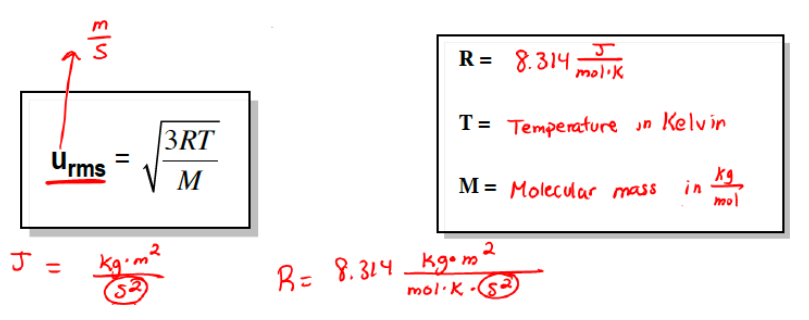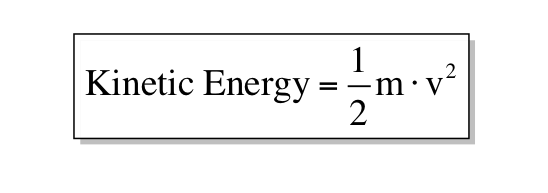Ch.1 - Intro to General Chemistry 2hrs & 53mins 0% complete Worksheet Ch.2 - Atoms & Elements 2hrs & 49mins 0% complete Worksheet Ch.3 - Chemical Reactions 3hrs & 25mins 0% complete Worksheet BONUS: Lab Techniques and Procedures 1hr & 38mins 0% complete Worksheet BONUS: Mathematical Operations and Functions 47mins 0% complete Worksheet Ch.4 - Chemical Quantities & Aqueous Reactions 3hrs & 30mins 0% complete Worksheet Ch.5 - Gases 3hrs & 47mins 0% complete Worksheet Ch.6 - Thermochemistry 2hrs & 28mins 0% complete Worksheet Ch.7 - Quantum Mechanics 2hrs & 35mins 0% complete Worksheet Ch.8 - Periodic Properties of the Elements 1hr & 57mins 0% complete Worksheet Ch.9 - Bonding & Molecular Structure 2hrs & 5mins 0% complete Worksheet Ch.10 - Molecular Shapes & Valence Bond Theory 1hr & 31mins 0% complete Worksheet Ch.11 - Liquids, Solids & Intermolecular Forces 3hrs & 40mins 0% complete Worksheet Ch.12 - Solutions 2hrs & 17mins 0% complete Worksheet Ch.13 - Chemical Kinetics 2hrs & 22mins 0% complete Worksheet Ch.14 - Chemical Equilibrium 2hrs & 26mins 0% complete Worksheet Ch.15 - Acid and Base Equilibrium 4hrs & 42mins 0% complete Worksheet Ch.16 - Aqueous Equilibrium 3hrs & 48mins 0% complete Worksheet Ch. 17 - Chemical Thermodynamics 1hr & 44mins 0% complete Worksheet Ch.18 - Electrochemistry 2hrs & 58mins 0% complete Worksheet Ch.19 - Nuclear Chemistry 1hr & 33mins 0% complete Worksheet Ch.20 - Organic Chemistry 3hrs 0% complete Worksheet Ch.22 - Chemistry of the Nonmetals 2hrs & 1min 0% complete Worksheet Ch.23 - Transition Metals and Coordination Compounds 1hr & 54mins 0% complete Worksheet

# Root Mean Square Speed

See all sections

Increasing the temperature allows a gas to absorb thermal energy and convert into kinetic energy. Kinetic energy allows the gas to move and the speed at which it moves gives us the root mean square speed

###### Root Mean Square Speed & Kinetic Energy

Concept #1: Understanding kinetic energy & Root Mean Square Speed

Transcript

Welcome back guys. In this new video, we're going to take a look at the kinetic energy of gases. We've talked about kinetic energy really quickly in previous videos. We say that when we increase the temperature of any type of container, the gas particles in there will absorb that thermal energy and convert it to kinetic energy. This is the energy that they're going to use in order to push themselves around inside of this container.
We're going to say in order to measure the average kinetic energy of a gas molecule or particle, we must employ the root mean square equation. The root mean square equation is U, which stands for velocity, equals square root of 3RT over M. When I say velocity, I mean speed. The units for velocity or speed are meters per second.
Here this R is not the same R we're used to seeing. Because we're talking about speed or energy, R is now 8.314 joules over moles times K. Remember when do I use this R? I use this R anytime we're talking about speed, velocity, kinetic energy. Energy is the key word here. The three keywords we look out for are energy speed or velocity. That's when we use this R. The other R is associated with the Ideal Gas Law. It's different.
Here, T represents temperature in Kelvin. Here, capital M means molar mass or molecular mass. But here it's different. We're used to seeing molecular mass or molar mass in grams per mole, but in this case, in this equation, it's going to be in kilograms per mole. You have to remember that. It's in kilograms per mole.
Now the R we're going to look at it a little bit closer. We're going to say here joules is just a form of energy. We're going to say joules are kilograms times meters squared over seconds squared. That's what joules mean. That means that our R is really 8.314 kilograms times meters squared over moles times K times seconds squared. Because the second squared is on the bottom here, we would have to put it on the bottom here. Just remember the units that are involved with joules. This is going to help us in our calculations to see what we isolate when we find our answer.Example #1: A 1.56 x 1013 pg gaseous particle travels at 6.21 m/s. Determine its kinetic energy.

The kinetic energy (in J or kJ) of a gas molecule is directly proportional to its absolute temperature in Kelvins.Practice: Calculate the molar mass, in g/mol, of a gaseous compound with an average root mean velocity of 652 m/s at a temperature of 30C.

Remember that using the root mean square speed equation deals with molar mass in g/mol, so further conversion may sometimes be needed.